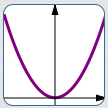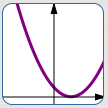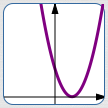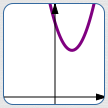﻿ Multi-Step Practice with all the Graphical Transformations

# MULTI-STEP PRACTICE WITH ALL THE GRAPHICAL TRANSFORMATIONS

• PRACTICE (online exercises and printable worksheets)

This lesson gives practice with all the graphical transformations:

Before practicing the multi-step exercises on this page, you should practice single-step transformations, all mixed up:
When you're successfully answering all the question types, then you're ready for this current lesson!

## EXAMPLE: Graphing Using Transformations

Graph   $\,y = 3(x-2)^2 + 5\,$   by starting with a ‘basic model’ and successively applying graphical transformations.

EQUATION ACTION GRAPHICAL RESULT (WORDS) GRAPHICAL RESULT
(cumulative graph)
$y = x^2$ the ‘basic model’ (the starting graph)start with the graph of the squaring function
$y = (x-1)^2$ replace every $\,x\,$ by $\,x-1\,$ right $\,1\,$when applying a transformation
that changes the $\,x\,$-values of points,
use the phrase ‘replace every $\,x\,$ by ...’
$y = 3(x-1)^2$ multiply previous $\,y\,$-values by $\,3\,$ vertical stretch,
$\,(a,b) \mapsto (a,3b)\,$when applying a transformation
that changes the $\,y\,$-values of points,
use the language ‘do something to previous $\,y\,$-values’;

the notation ‘$\,(a,b) \mapsto (a,3b)\,$’ is read as
‘$\,(a,b)\,$ maps to $\,(a,3b)\,$’;
it clearly shows that the $\,y\,$-values of previous points
are getting multiplied by $\,3\,$
$y = 3(x-1)^2 + 5$ add $\,5\,$ to previous $\,y\,$-values up $\,5\,$Other orders will work. For example:

 $y = x^2$ (entire sequence, lightest to darkest)$y = 3x^2$ $y = 3x^2 + 5$ $y = 3(x-1)^2 + 5$

## PRACTICE: Given the Desired Graphical Results, Produce the Equations

 Give the equations and actions that produce the successive desired graphical results. Check your answers by clicking the ‘SHOW ANSWER’ buttons. EQUATION ACTION GRAPHICAL RESULT
Master the ideas from this section